Function Repository Resource:

# PermutationGraph

Compute the permutation graph of a permutation

Contributed by: Wolfram Staff (original content by Sriram V. Pemmaraju and Steven S. Skiena)
 ResourceFunction["PermutationGraph"][p] gives the permutation graph for the permutation p.

## Details and Options

Vertices of a permutation graph represent the elements of a permutation; edges represent pairs of elements that are inversions in the permutation.
ResourceFunction["PermutationGraph"] accepts the same options as Graph.

## Examples

### Basic Examples (1)

A permutation Graph of a permutation:

 In:=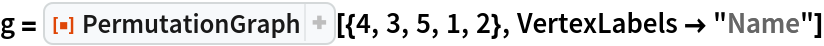Out=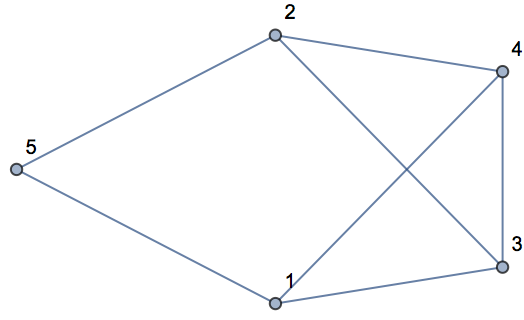### Properties and Relations (3)

GraphComplement gives the permutation graph of the reverse permutation p:

 In:=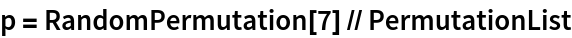Out=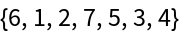In:=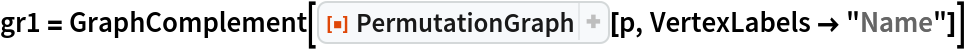Out=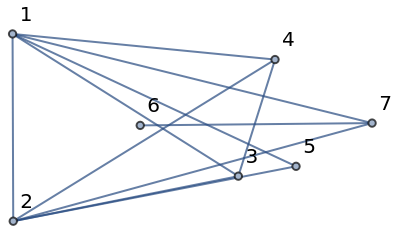In:=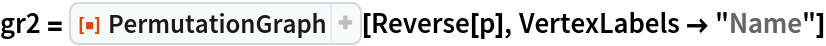Out=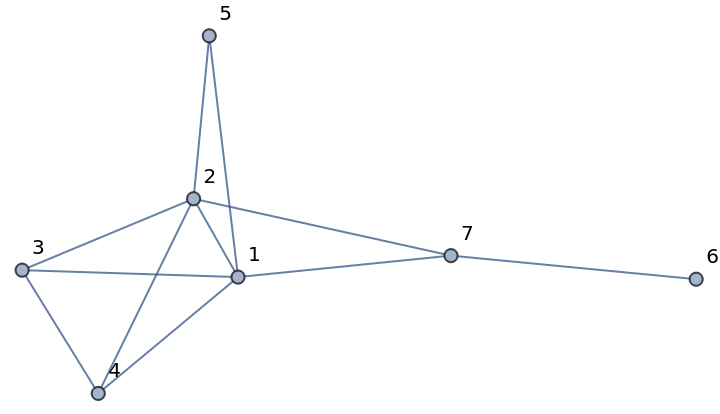In:=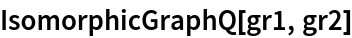Out=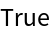The number of inversions in a permutation can be computed by the resource function InvesionCount and is equal to the number of edges in its permutation graph:

 In:=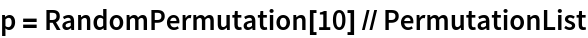Out=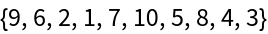In:=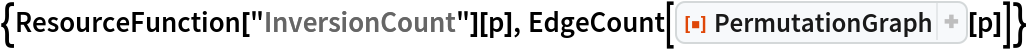Out=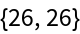Every clique in a permutation graph corresponds to a decreasing sequence in the corresponding permutation:

 In:=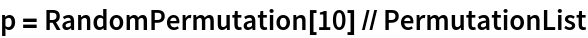Out=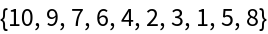In:=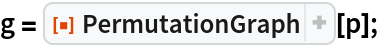In:=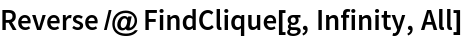Out=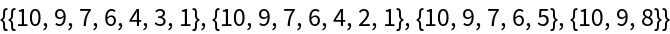A maximum-size clique corresponds to one of the longest decreasing sequences:

 In:=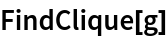Out=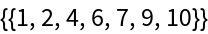In:=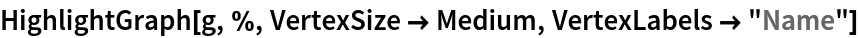Out=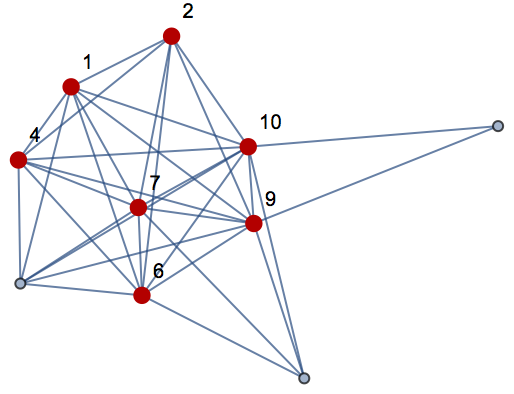## Version History

• 1.0.0 – 07 July 2020